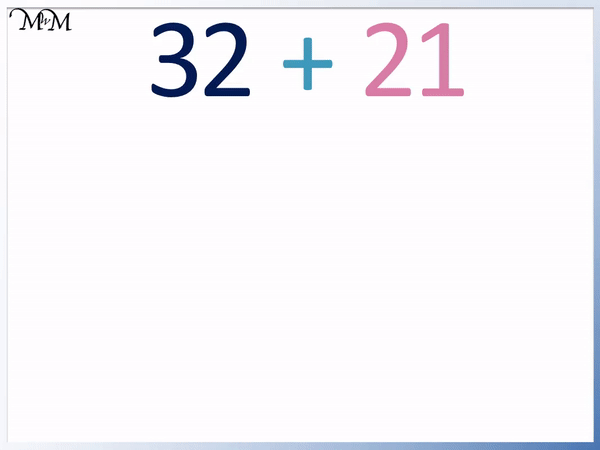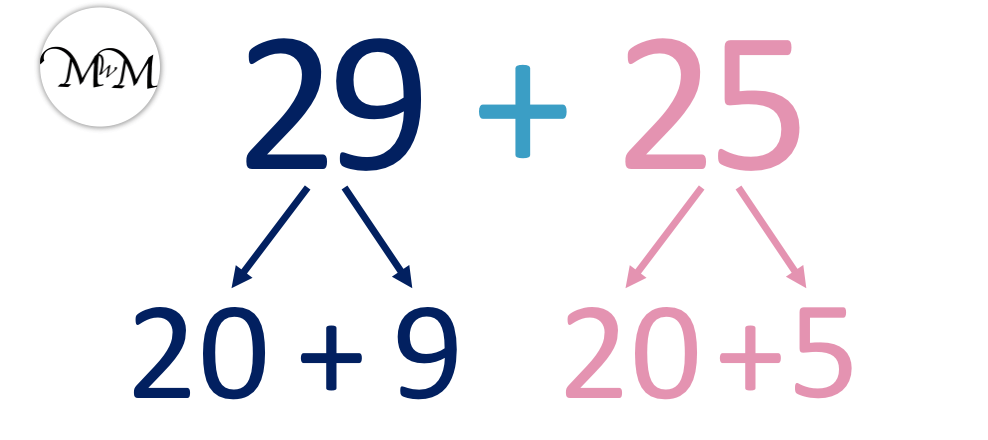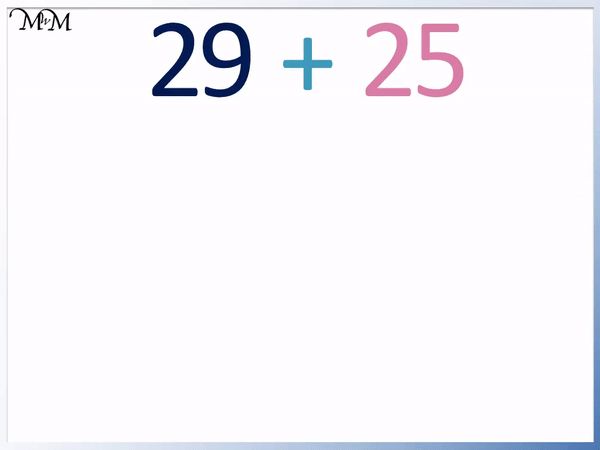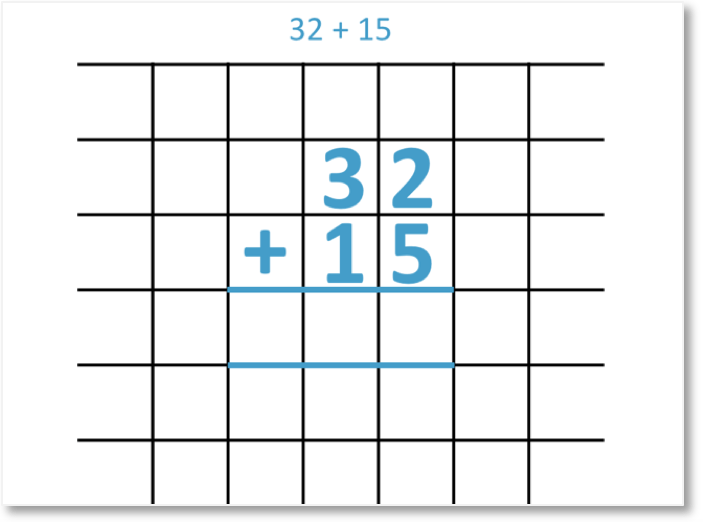• We partition the two numbers by writing them as the sum of their tens and units.
• Multiply the tens digit in each number by ten to see its value.
• For example, 32 = 30 + 2 and 21 = 20 + 1.
• We add the tens and the units separately.
• We can see that 30 + 20 = 50 because 3 + 2 = 5.
• Adding the units, 2 + 1 = 3.
• Once we have added the tens and units separately, we add the two results together.
• 50 + 3 = 53 and so, 32 + 21 = 53.
• Addition using the partitioning strategy is also known as the split strategy for addition.

Split each number into its tens and units.

Add the tens and units separately and then add these results to find the total.• We first split the numbers into their tens and units.
• 63 contains 6 tens and 3 units and so, 63 = 60 + 3.
• 17 contains 1 ten and 7 units and so, 17 = 10 + 7.
• We add the tens in each number.
• 60 + 10 = 70.
• Next we add the units.
• 3 + 7 = 10.
• Finally, we add these results.
• 70 + 10 = 80 and so, 63 + 17 = 80.# Addition using the Partitioning Strategy

## What is the Partitioning Strategy for Addition?

The partitioning strategy is also known as the split strategy. The partitioning strategy is a method used to break down larger additions into smaller additions that are easier to do.

Addition by partitioning involves splitting numbers into their hundreds tens and units. The hundreds, tens and units are added separately.

Addition by partitioning is useful because it provides us with an alternative strategy for adding numbers. It is a particularly important strategy for mental addition. This process can be learnt as shown in this lesson by writing out the steps and eventually, when this is method is mastered, addition by partitioning can be done mentally.

Addition by partitioning is one of the most effective strategies for mental addition.

For example, here is the addition of 32 + 21.

The addition is easier to work out by breaking it down into steps.32 contains 3 in the tens column and 2 in the ones column.

3 tens are 30. We simply put a zero digit on the end of the 3.

32 can be split into 30 + 2.

21 is made up of 2 tens and 1 one.

21 can be split into 20 + 1.

Splitting the numbers into their tens and ones is called partitioning. We can partition numbers in other ways. For example we can partition 32 into 28 + 4 but this is not a helpful way to break down the addition.

In the split strategy, we always partition the numbers into tens and ones.Once both numbers have been partitioned, we add the tens of one number to the tens of the other number.

We have 30 + 20. Adding the tens is simple because we can treat this as 3 + 2 but with a zero on the end.

30 + 20 = 50 because 3 + 2 = 5. The sum of the tens is 50.

We now add the ones. We have 2 + 1 = 3. The sum of the ones is 3.

Once we have added the tens and the ones, all that remains is to find the total to get our answer. We add the sum of the tens to the sum of the ones.

50 + 3 = 53 and so, 32 + 21 = 53.

## Addition using the Partitioning Strategy

To add numbers using the partitioning strategy, use the following steps:

• Write each number as the sum of the values of its digits.
• Add the place value columns of each number together.
• For example, add the hundreds of each number together.
• Add the tens of each number together.
• Add the ones of each number together.

For example, here is 45 + 32. We will use the addition by partitioning method (or split strategy) to work this out.The first step is to look at each number and see that they are made up of a tens digit and a one digit.

45 is 40 + 5 and 32 is 30 + 2.

There are no hundreds to add in this number but we have tens and units.

We add the tens first. We have 40 and 30.40 + 30 = 70

5 + 2 = 7

Having added the tens and ones separately, the final step is to now add these two answers together.

The sum of the tens is 70 and the sum of the ones is 7.

70 + 7 = 77

Therefore, 45 + 32 = 77.

We will use the split strategy in this next example of 29 + 25.

The first step is to partition the numbers into their tens and ones.

29 = 20 + 9 and 25 = 20 + 5.20 + 20 = 40

And now we add the ones.

9 + 5 = 14